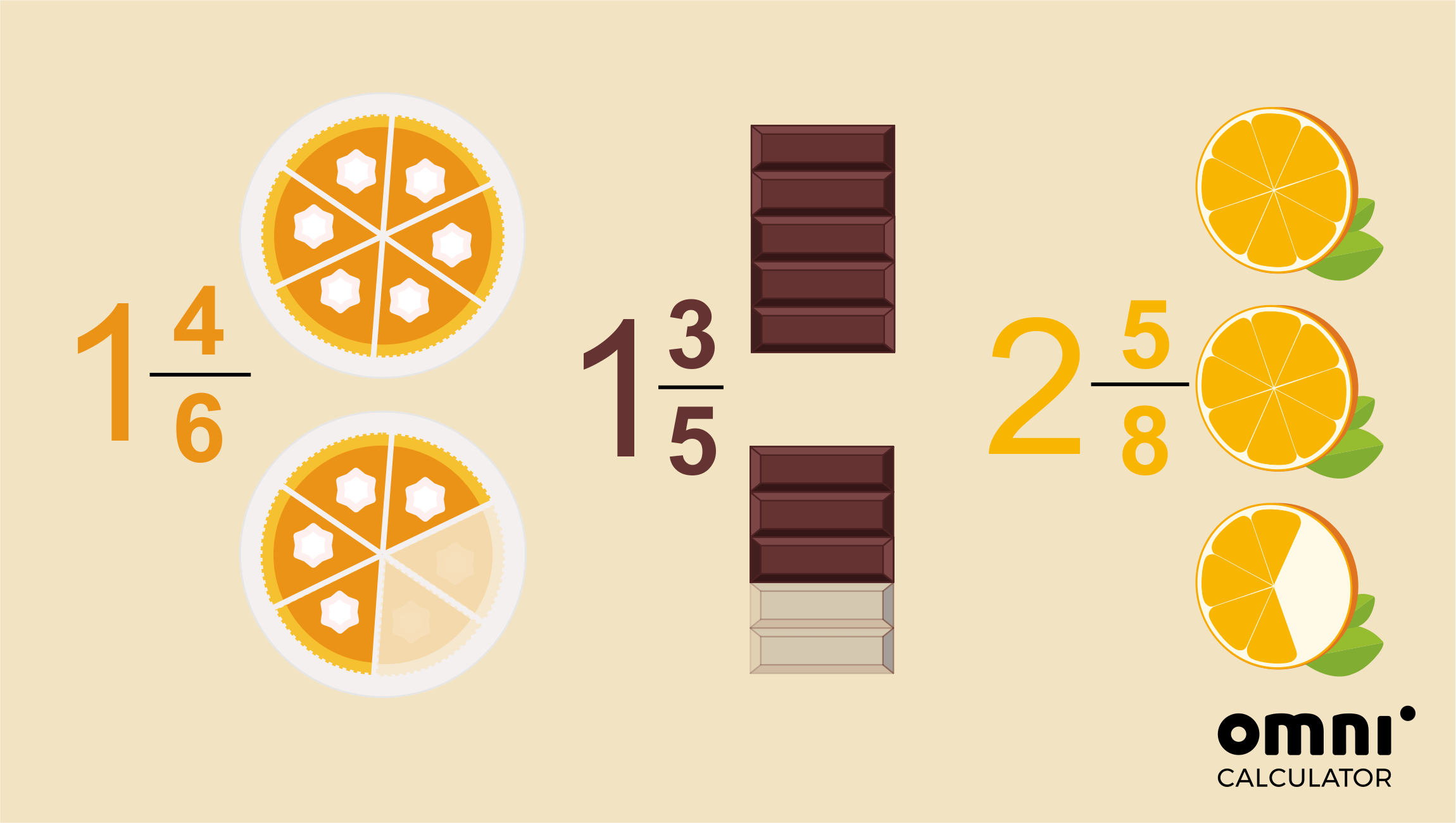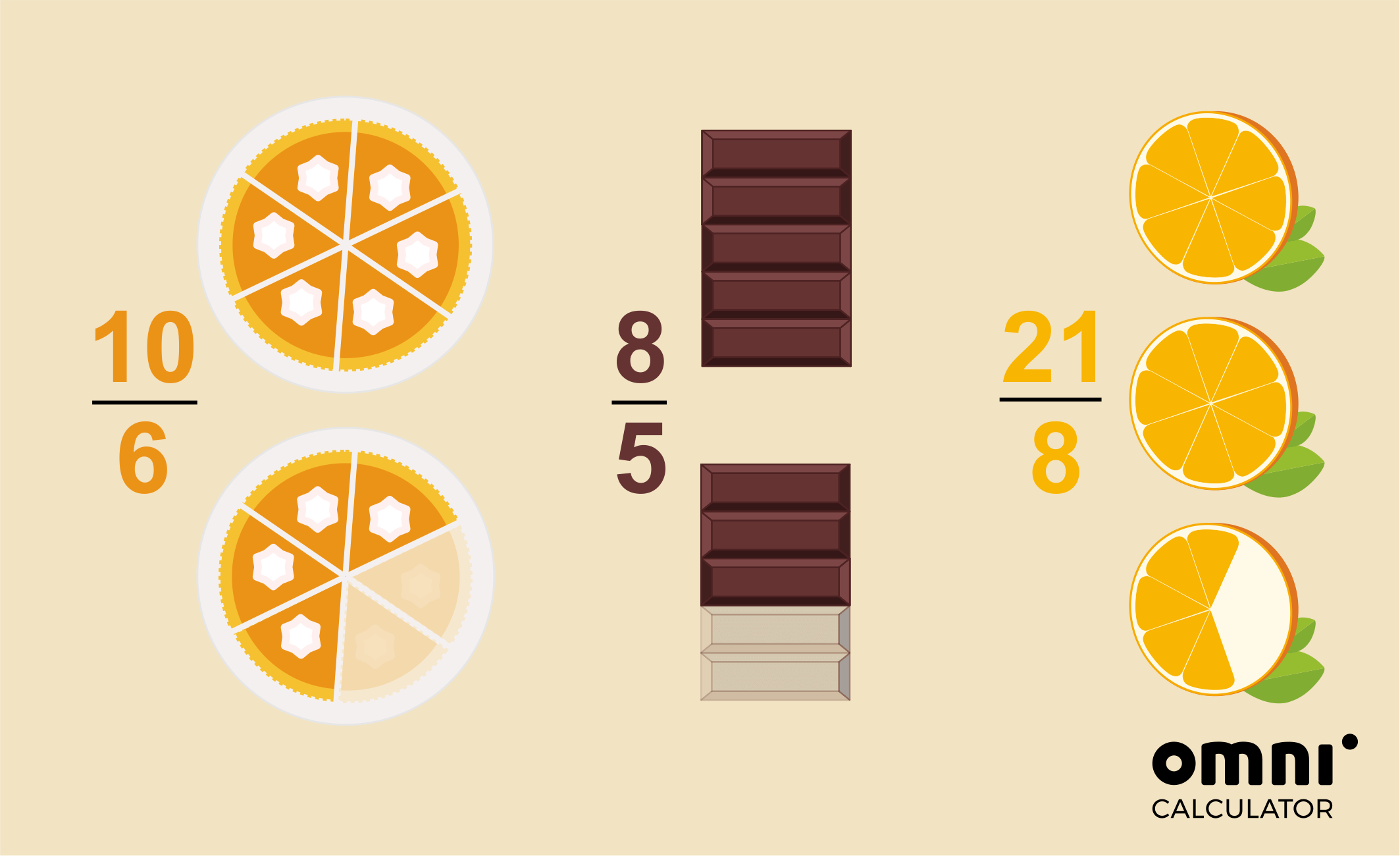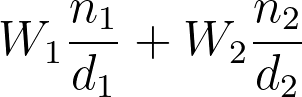# Mixed Number Calculator

Created by Hanna Pamuła, PhD candidate
Reviewed by Dominik Czernia, PhD candidate and Jack Bowater
Last updated: Apr 06, 2022

This mixed number calculator (also a mixed fraction calculator) will help you solve any issues with mixed numbers:

Adding and subtracting mixed fractions? Here it is✔️

Multiplying and dividing mixed numbers? Of course!✔️

Simplifying mixed fractions? You're covered✔️

Mixed number to decimal and decimal to mixed number conversions? Right here!✔️

That is power of the mixed number calculator.

Scroll down to learn what a mixed number is. After you have found the mixed number definition, you can then read more about the operations you can do to mixed fractions. However, if this is the start of your journey into the world of fractions, the versatile fraction calculator is a must!

## What is a mixed number? Mixed number definition

A mixed number, also called a mixed fraction, is one of the ways to represent a fraction. In mixed fractions, you have a whole number and a proper fraction put together - and that's where the name comes from, you're actually mixing two types of numbers!
Examples of the mixed fractions are:

🍰 1 whole cake and 4 slices out of 6 of another → 1 4/6

🍫 1 whole chocolate bar and an additional 3 rows out of 5→ 1 3/5

🍊 2 whole oranges and 5 segments out of 8 from one more → 2 5/8There's also another representation which can show the same amount - an improper fraction. Look at the image again - what will happen if you add all equal slices together, instead of taking the whole items separately?That's how improper fractions work - they show how many parts you have, and they don't specify if you have whole items. It's still the same number, just written differently!

Now that you know the mixed number definition, read the on how to turn a fraction into a mixed number (and vice versa).

## How to change a mixed number to a improper fraction? How to turn a fraction into a mixed number?

In this section, we'll show you how to convert between mixed numbers and improper fractions.

• Mixed number to an improper fraction, e.g. 3 1/4
1. Multiply the whole number by the denominator: 3 * 4 = 12
2. Add the result to your numerator: 12 + 1 = 13
3. That's your new numerator - write it on top of your denominator: 13/4

• Improper fraction to a mixed number, e.g. 17/6
1. Divide the numerator by the denominator (the top number by the bottom number): 17 / 6 = 2 remainder 5
2. Take the whole number from the division - it's the whole part of the mixed number: 2
3. Find the fractional part: the remainder from the division is the new numerator, the denominator stays the same as in the original fraction: 5/6
4. Put two numbers together - that's your mixed number: 25/6

Note that what we present here is just a condensed version of the step by step instructions - if you need a more thorough explanation, check out our dedicated improper fraction to mixed number tool and mixed number to improper fraction calculator.

## Adding mixed numbers

There are two popular ways of adding mixed numbers. Let's check it with the example of adding 2 2/5 and 1 1/2

Method 1: adding whole numbers and fractions separately

As the number is mixed, you can do the adding operation separately on the whole part and fractional part:

• At first, add whole numbers:

2 + 1 = 3

• Next, add the two fractional parts. In our case the fractions have uneven denominators, so you need to find their least common multiple:

LCM(2,5) = 10

2/5 + 1/2 = 4/10 + 5/10 = 9/10

• Put the two parts together:

3 9/10

For our example that's all you need to do, but it may happen that adding fractions will result in an improper fraction - then, you'll need to change it into a mixed number. Take a look at an example - adding 2 3/5 and 1 1/2:

2 3/5 + 1 1/2

2 + 1 = 3

3/5 + 1/2 = 6/10 + 5/10 = 11/10 = 1 1/10

So 3 + 1 1/10 = 4 1/10

Method 2: Converting mixed numbers to improper fractions

• Let's start by converting our mixed numbers to their equivalent improper fractions:

2 2/5 = 12/5

1 1/2 = 3/2

• Do the standard addition of fractions with uneven denominators:

12/5 + 3/2 = 24/10 + 15/10 = 39/10

• Finally, convert your result back into a mixed number:

39/10 = 3 9/10

In our mixed number calculator, we've implemented the latter method. Give it a go!

## Subtracting mixed fractions

Subtracting mixed fractions is similar to adding mixed numbers: all in all, the only the difference is the sign.

However, things may become a little bit more complicated in certain special cases: if the fractional part of the first mixed number is smaller than the fractional part of the second mixed fraction. In such a case, you should regroup the whole and fractional part, which may be a bit confusing for some people (check how regrouping works with this ).

To avoid this problem, use the second method for subtracting mixed fractions - that is changing your mixed fractions to improper fractions. This technique will ensure that the steps are the same, no matter what the fractions are.

## Multiplying mixed fractions

To multiply two mixed numbers, you should again use the mixed number ↔ improper fraction conversion. Let's transform a multiplying mixed fractions concept into a word problem:

You're a big fan of a pumpkin pie 🥧 If you could, you would eat a whole pie every day. Unfortunately, your mum doesn't let you (well, she knows what's good for your health, right? 💪). She imposed the rule that you can eat only one and a half pumpkin pies per week - and you can have an equal slice of the pie every day. The question is, how many pies would you eat in two weeks and five days - more or less than four?

Let's have a look at the step-by-step instruction and solution:

1. Change your mixed numbers to improper fractions:

You can write one and a half pie as 1 1/2:
1 1/2 = 3/2,

and two weeks and five days as 2 5/7: 2 5/7 = 19/7.

1. Multiply the numerator by the other numerator and the denominator by the other denominator:

3/2 * 19/7 = (3 * 19)/(2 * 7) = 57/14

1. Change the result into a mixed fraction:

57/14 = 4 1/14

Ooops. That's more than four pies! Type the numbers into the mixed number calculator to verify the result.

If you are on the hunt for more examples and instructions on how to multiply fractions with mixed numbers or whole numbers, make sure to look at our comprehensive multiplying fractions calculator.

## Dividing mixed numbers

We will explain division as quickly as possible - dividing mixed numbers is very similar to multiplying mixed fractions. Only one additional step is needed - to get the reciprocal of your second fraction (divisor). If you don't know what reciprocal means, its just flipping the fraction upside down. If you wanted to divide mixed numbers 1 1/2 and 2 5/7, you would:

1. Change the mixed numbers to improper fractions: 1 1/2 = 3/2, 2 5/7 = 19/7
2. Find the reciprocal of the second fraction:  19/7 → 7/19
3. Multiply the fractions (the numerator by the other numerator and the denominator by the other denominator):

3/2 * 7/19 = (3 * 7)/(2 * 19) = 21/38

## Mixed number to decimal & decimal to mixed number

Ok, we have to confess - we're sometimes a bit lazy 🦥 And that's the case here: we don't want to repeat ourselves too much! Because why should we write the same thing again and again if we've already done it? 🤔 Check out our other awesome calculators and read the detailed explanations on mixed number to decimal or decimal to mixed number conversions!

• The decimal to fraction calculator has a great section on how to turn any fraction into a decimal. Of course, the instruction also works for mixed number to decimal conversion 🎉
• Our fraction to decimal calculator presents two different methods on how to deal with fractions to decimal conversions - and mixed number to decimal is no exception. Pop in and have a good read! 🔖
Hanna Pamuła, PhD candidate
I want to
➕ add mixed numbers1st fraction
Whole number (W₁)
Numerator (n₁)
Denominator (d₁)
2nd fraction
Whole number (W₂)
Numerator (n₂)
Denominator (d₂)
Result
If you want to add more than two fractions, or want a step by step solution, check out our adding fractions calculator.
People also viewed…

### Body fat

Use the body fat calculator to estimate what percentage of your body weight comprises of body fat.

### Coffee kick

A long night of studying? Or maybe you're on a deadline? The coffee kick calculator will tell you when and how much caffeine you need to stay alert after not sleeping enough 😀☕ Check out the graph below!

### Parallel line

Parallel line calculator finds the equation of a straight line parallel to a given line, as well as the distance between them.

### Power set

The power set calculator is here to list and count the number of subsets of a set with up to ten elements.